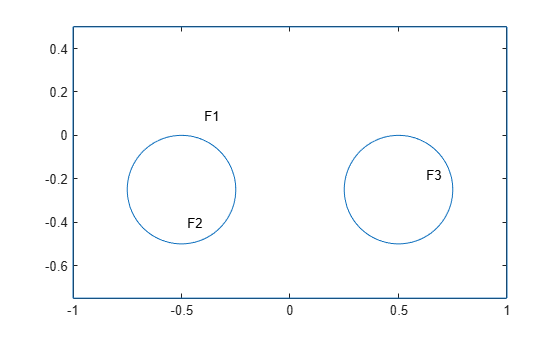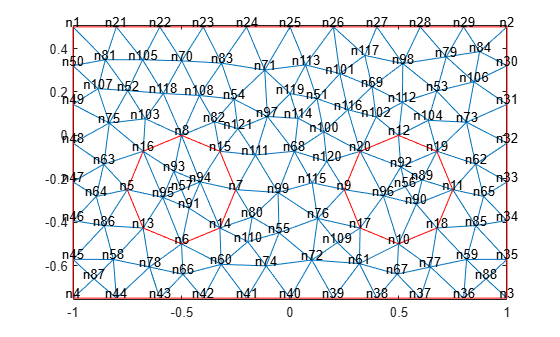# pdesdp

(Not recommended) Indices of subset of mesh nodes belonging to specified faces of 2-D geometry

`pdesdp` is not recommended. Use `findNodes` instead.

## Syntax

``s = pdesdp(p,e,t,FaceID)``
``[i,c] = pdesdp(p,e,t,FaceID)``
``___ = pdesdp(p,e,t)``

## Description

example

````s = pdesdp(p,e,t,FaceID)` returns the indices of the nodes of the `[p,e,t]` mesh shared between two or more faces listed in `FaceID`.```

example

````[i,c] = pdesdp(p,e,t,FaceID)` returns the indices of the nodes of the `[p,e,t]` mesh belonging strictly to faces `FaceID` as `i`. It also returns the indices of the nodes shared between `FaceID` and faces not listed in `FaceID` as `c`.```
````___ = pdesdp(p,e,t)` uses any of the previous syntaxes, assuming that `FaceID` is a list of all faces of a 2-D geometry.```

## Examples

collapse all

Find the indices of the mesh nodes belonging strictly to the specified faces and the indices of the nodes shared between faces.

Define two circles and a rectangle and place these in one matrix.

```R1 = [3,4,-1,1,1,-1,0.5,0.5,-0.75,-0.75]'; C1 = [1,-0.5,-0.25,0.25]'; C2 = [1,0.5,-0.25,0.25]'; C1 = [C1;zeros(length(R1) - length(C1),1)]; C2 = [C2;zeros(length(R1) - length(C2),1)]; gd = [R1,C1,C2];```

Create a set formula that adds the circles to the rectangle.

`sf = 'R1+C1+C2';`

Create and plot the geometry.

```ns = char('R1','C1','C2'); ns = ns'; gd = decsg(gd,sf,ns); pdegplot(gd,'FaceLabels','on')```Create a mesh.

`[p,e,t] = initmesh(gd);`

Plot the mesh with the node labels.

`pdemesh(p,e,t,'NodeLabels','on')`Find the indices of the mesh nodes shared between faces 1 and 2.

`s1 = pdesdp(p,e,t,[1 2])`
```s1 = 1×8 5 6 7 8 13 14 15 16 ```

Find the indices of the mesh nodes shared between faces 2 and 3. Since these faces do not share any nodes, `pdesdp` returns an empty vector.

`s2 = pdesdp(p,e,t,[2 3])`
```s2 = 1x0 empty double row vector ```

Find the nodes belonging strictly to face 2 and also the nodes shared between face 2 and other faces. Face 2 shares nodes only with face 1, therefore, vectors `c` and `s1` consist of the same face IDs.

`[i,c] = pdesdp(p,e,t,2)`
```i = 1×5 56 88 90 91 92 ```
```c = 1×8 5 6 7 8 13 14 15 16 ```

## Input Arguments

collapse all

Mesh nodes, specified as a 2-by-`Np` matrix of nodes (points), where `Np` is the number of nodes in the mesh. For details on the mesh data representation, see `initmesh`.

Data Types: `double`

Mesh edges, specified as a `7`-by-`Ne` matrix of edges, where `Ne` is the number of edges in the mesh. For details on the mesh data representation, see `initmesh`.

Data Types: `double`

Mesh elements, specified as a `4`-by-`Nt` matrix of triangles, where `Nt` is the number of triangles in the mesh. For details on the mesh data representation, see `initmesh`.

Data Types: `double`

Face IDs, specified as a vector of integers.

Data Types: `double`

## Output Arguments

collapse all

Mesh nodes shared between two or more specified faces, returned as a row vector of positive integers representing the indices of the nodes.

Mesh nodes belonging only to specified faces, returned as a row vector of positive integers representing the indices of the nodes.

Mesh nodes shared between specified and other faces, returned as a row vector of positive integers representing the indices of the nodes.

## Version History

Introduced before R2006a

expand all